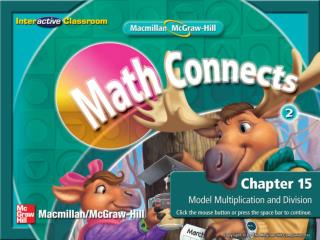DownloadDownload PresentationSplash Screen

# Splash Screen

Download Presentation## Splash Screen

- - - - - - - - - - - - - - - - - - - - - - - - - - - E N D - - - - - - - - - - - - - - - - - - - - - - - - - - -
##### Presentation Transcript

1. Splash Screen

2. Are You Ready for Chapter 15? • Lesson 1: Multiplication Stories • Lesson 2: Equal Groups • Lesson 3: Problem-Solving Strategy: Draw a Picture • Lesson 4: Repeated Addition • Lesson 5: Arrays • Lesson 6: Division Stories • Lesson 7: Find Equal Groups • Lesson 8: Problem-Solving Investigation: Choose a Strategy • Chapter 15 Review Select a hyperlink to go to the corresponding slides. Chapter Menu

8. Introduction—End

9. I will model and create multiplication stories. Lesson 1—Get Ready 1

10. multiplication Lesson 1—Get Ready 2

12. Use counters to model this multiplication story: • I have 3 different flavors of ice cream. There are 5 scoops of each flavor. How many scoops of ice cream are there in all? Lesson 1—Teach

13. There are 3 flavors, so there are 3 groups. There are 5 scoops in each group. Lesson 1—Teach

14. How many scoops are there in all? 15 scoops Lesson 1—Teach

15. There are 3 bird feeders. 2 birds are on each bird feeder. How many birds are there in all? 6 birds Lesson 1—Check

16. Lesson 1—End

17. I will model skip counting to find the total in equal groups. Lesson 2—Get Ready 1

18. equal groups Lesson 2—Get Ready 2

21. 1 2 3 4 5 6 7 8 9 10 How many equal groups of 2 counters can you make? 10 equal groups Lesson 2—Teach

22. 2 4 6 8 10 12 14 16 18 20 • Find the total number of counters by skip counting by twos. 20 total counters Lesson 2—Teach

23. 1 2 3 4 5 • How many equal groups of 4 counters can you make? 5 equal groups Lesson 2—Teach

24. 12 16 20 4 8 • Find the total number of counters by skip counting by fours. 20 total counters Lesson 2—Teach

25. How many equal groups of 3 are there? 4 groups of 3 Lesson 2—Check

26. Skip count. Write how many hearts in all. 12 hearts Lesson 2—Check

27. How many equal groups of 2 are there? 5 groups of 2 Lesson 2—Check

28. Skip count. Write how many suns in all. 10 suns Lesson 2—Check

29. Lesson 2—End

30. I will draw a picture to solve a problem. Lesson 3—Get Ready 1

32. Lesson 3—Teach

33. What do I know? Underline what you know. Lesson 3—Teach

34. What do I need to find? Circle the question. Lesson 3—Teach

35. How will I solve the problem? I will draw a picture. Lesson 3—Teach

36. Draw a picture. 5 wreaths 4 8 4 pinecones per wreath Brenda needs 20 pinecones in all. 12 16 20 Lesson 3—Teach

37. Look back.Is my answer reasonable? yes Lesson 3—Teach

38. Draw a picture to solve. • Teagan bought 4 bags of apples. Each bag had 4 apples in it. How many apples did Teagan buy in all? 16 apples Lesson 3—Check

39. Lesson 3—End

40. I will add and multiply equal groups. Lesson 4—Get Ready 1

41. multiplication sentence • product Lesson 4—Get Ready 2

43. How many equal groups of boxes are shown? 2 equal groups Lesson 4—Teach

44. How many boxes are in each group? 5 boxes Lesson 4—Teach

45. What repeated addition problem can we make from the picture? 5 + 5 = 10 Lesson 4—Teach

46. How would you write this repeated addition problem as a multiplication sentence? 2 × 5 = 10 Lesson 4—Teach

47. How many equal groups of boxes are shown? 3 equal groups Lesson 4—Teach

48. Write the repeated addition problem to show the picture. 4 + 4 + 4 = 12 Lesson 4—Teach

49. Write the multiplication sentence to show the picture. 3 × 4 = 12 Lesson 4—Teach

50. The first number tells how many groups there are. 3 × 4 = 12 Lesson 4—Teach